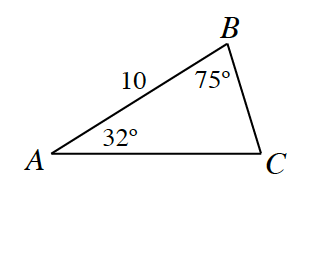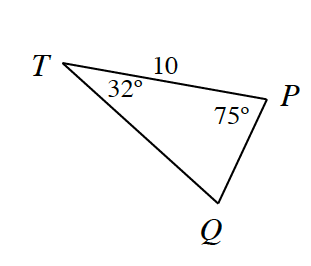### Home > INT1 > Chapter 11 > Lesson 11.2.6 > Problem11-128

11-128.

Are the two triangles below congruent? If so, give a congruence condition and a series of rigid transformations to justify your answer.These triangles are congruent by $ASA≅$. Translate point $T$ to point $A$, then rotate $ΔT'P'Q'$about point $T'$ so that $AB$ and $T''P''$ coincide.